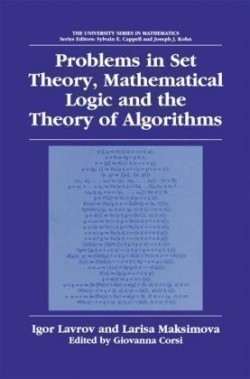# Problems in Set Theory, Mathematical Logic and the Theory of Algorithms

Lavrov, Igor; Springer, Berlin;Print on demand

# Problems in Set Theory, Mathematical Logic and the Theory of Algorithms

Lavrov, Igor; Springer, Berlin;
Book
CZK 3,159
Print on demand
Delivery on Monday, 17. of January 2022
CZK 3,159 with DPH
You save CZK 351 CZK 3,510 (-10%)
Problems in Set Theory, Mathematical Logic and the Theory of Algorithms by I. Lavrov & L. Maksimova is an English translation of the fourth edition of the most popular student problem book in mathematical logic in Russian. It covers major classical topics in proof theory and the semantics of propositional and predicate logic as well as set theory and computation theory. Each chapter begins with 1-2 pages of terminology and definitions that make the book self-contained. Solutions are provided. The book is likely to become an essential part of curricula in logic.
EAN
9781461349570
ISBN
1461349575
Bindings
Paperback / softback
Publisher
Springer, Berlin
Pages
282
Language
Angličtina
Dimensions
235 x 155